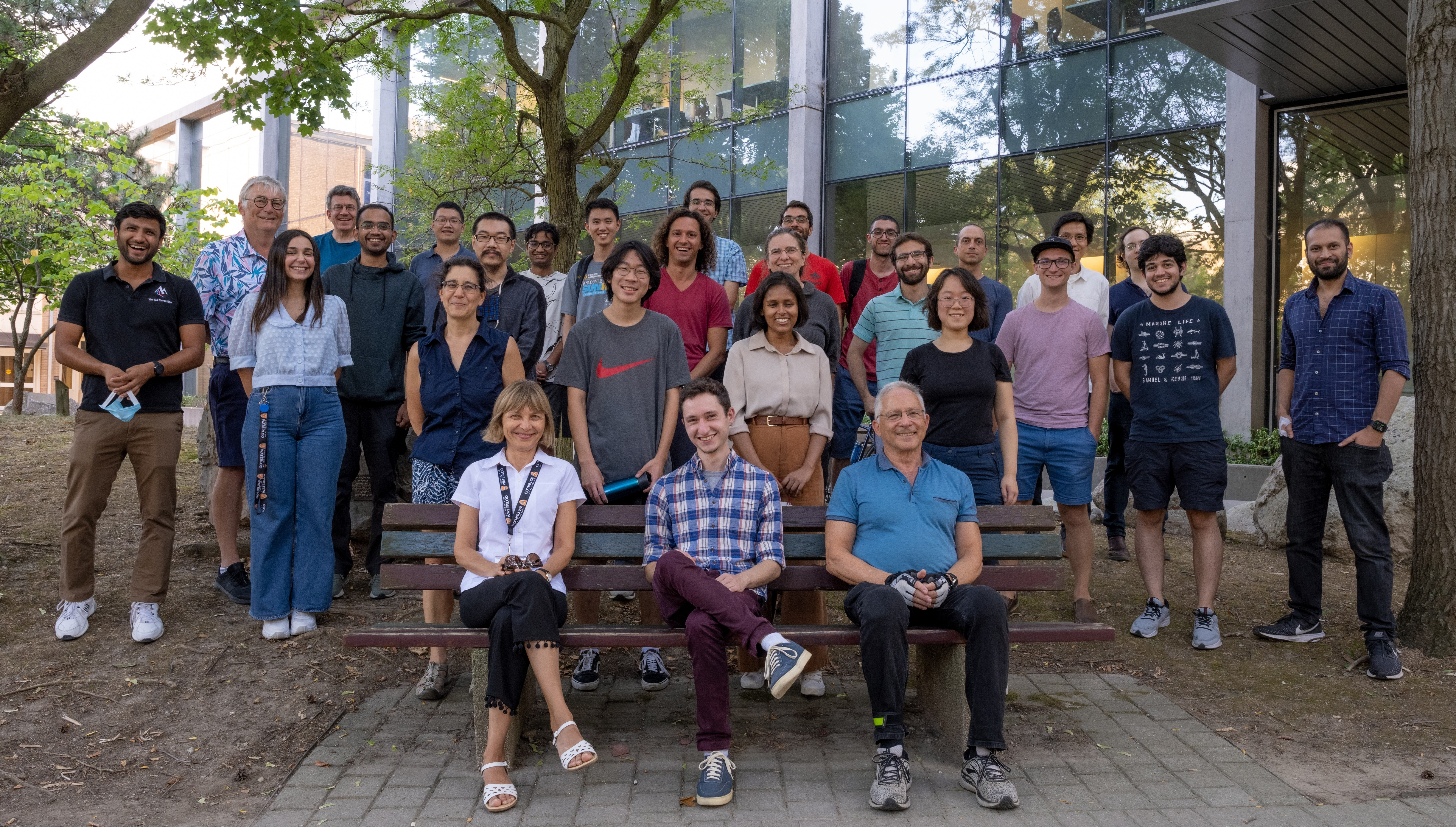# Algorithms & Complexity Group

The University of Waterloo is a wonderful place to conduct research in Theoretical Computer Science. The remarkable breadth of TCS research conducted in the Faculty of Mathematics by the A&C group, as well as the Combinatorics & Optimization, Cyptography and Symbolic Computation groups, coupled with active research groups in Pure Mathematics, Statistics and the Institute for Quantum Computing makes Waterloo an exciting place for exploring the limits of computation.

# Research Areas

• Algebraic Complexity
• Analysis of Algorithms
• Approximation Algorithms
• Automata Theory
• Bioinformatics
• Combinatorics on Words
• Complexity Theory
• Computational Algebra
• Computational Geometry
• Data Structures
• Differential Privacy
• Fixed Parameter Tractability
• Formal Languages
• Graph Drawing
• Graph Theory
• Learning Theory
• Machine Learning
• Network Routing
• Optimization
• Quantum Computation
• Quantum Information Theory
• Randomized Algorithms
• Spectral Graph Theory